# Step 3: Join Selection

The job of the query optimizer is incredibly complex. The optimizer can consider literally thousands of options when determining the optimal execution plan. The statistics are simply one of the tools that the optimizer can use to help in the decision-making process.

In addition to examining the statistics to determine the appropriate use of indexes, the optimizer must consider the optimum order in which to access the tables, the appropriate join algorithms to use, the appropriate sorting algorithms, and many other details that are too many to list here. The goal of the optimizer during join selection is to determine the lowest cost join strategy in terms of logical I/Os and the amount of memory required.

As mentioned at the beginning of this chapter, delving into the detailed specifics of the various join strategies and their costing algorithms is beyond the scope of a single chapter on optimization. In addition, some of these costing algorithms are proprietary and not publicly available.

The goal of this section then is to present an overview of the more common query processing algorithms that the optimizer uses to determine an appropriate execution plan.

#### Join Processing Strategies

If you are familiar with SQL, you are probably very familiar with using joins between tables in creating SQL queries. As far as the SQL Server query processor is concerned , a join occurs anytime it has to compare two inputs to determine an output. The join can occur between one table and another table, between an index and a table, or between an index and another index (as you've seen previously in the section on index intersection).

The SQL Server query processor uses three basic types of join strategies when it must compare two inputs: nested loops join; merge join; and hash join. The optimizer must consider each one of these algorithms to determine the most appropriate and efficient algorithm for a given situation.

Each of the three supported join algorithms could be used for any join operation. The query optimizer examines all the possible alternatives, assigns costs to each, and chooses the least expensive join algorithm for a given situation. The addition of the merge and hash joins in SQL Server 7.0 has greatly improved the query processing performance of SQL Server in the data warehousing and very large database environment. In previous versions that supported only nested loops join strategies, SQL Server was not as effective at handling large data requests .

##### Nested Loops Join

The nested loops join algorithm is by far the simplest of the three supported algorithms. The nested loops join uses one input as the "outer" loop and the other input as the "inner" loop. As you might expect, SQL Server processes the outer input one row at a time. For each row in the outer input, the inner input is searched for matching rows.

Figure 35.12 illustrates a query that uses a nested loops join.

##### Figure 35.12. Execution plan for a nested loops join.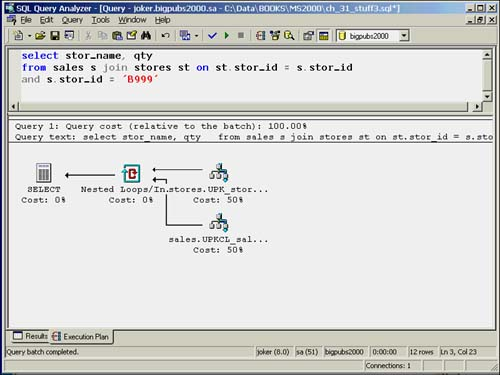Note that in the graphical showplan, the outer loop is represented as the top input table and the inner loop is represented as the bottom input table. In most instances, the optimizer will choose the input table with the fewest number of qualifying rows to be the outer loop.

The nested loops join is efficient for queries that typically affect only a small number of rows. As the number of rows in the outer loop increases , the effectiveness of the nested loops join strategy diminishes. This is because of the increased number of logical I/Os required as the loops get larger.

The nested loop join is the easiest join strategy to estimate the cost for. The cost of the nested loop join is calculated as follows :

number of pages to read in outer input

+ number of matching rows x number of pages per lookup on inner input

= total logical I/O cost for query

##### Merge Join

The merge join algorithm is much more effective than the nested loops join when dealing with large data volumes . The merge join works by retrieving one row from each input and comparing them. Figure 35.13 illustrates a query that will use a merge join.

##### Figure 35.13. Execution plan for a merge join.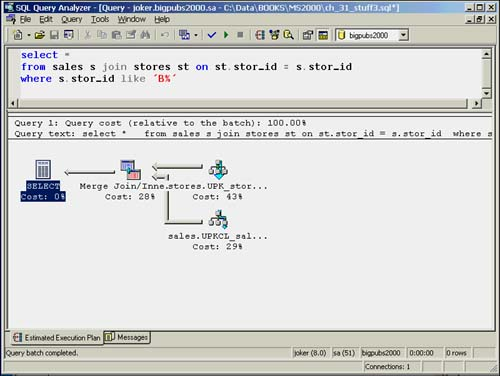The merge join requires that both inputs be sorted on the merge columns . The merge join does not work if both inputs are not sorted. In the query shown in Figure 35.13, both tables have a clustered index on stor_id, so the merge column (stor_id) is already sorted for each table.

Usually, the optimizer will choose a merge join strategy, as it did in this example, when the data volume is large and both columns are contained in an existing presorted index, such as a primary key. If either of the inputs is not already sorted, the optimizer has to perform an explicit sort before the join. An example of a sort being performed before the merge join is performed is shown in Figure 35.14.

##### Figure 35.14. Execution plan for a merge join with a preliminary sort step.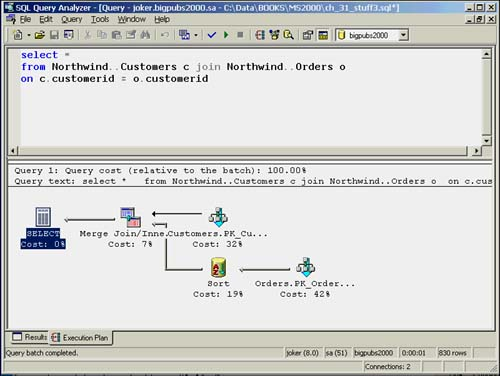If one or more of the inputs is not sorted, and the additional sorting causes the merge join to be too expensive to perform, the optimizer might consider using the hash join strategy.

##### Hash Join

The final ”and most complicated ”join algorithm is the hash join. The hash join is an effective join strategy for dealing with large data volumes where the inputs might not be sorted and when no useful indexes exist on your tables for performing the join. Figure 35.15 illustrates a query that uses a hash join.

##### Figure 35.15. Execution plan for a hash join.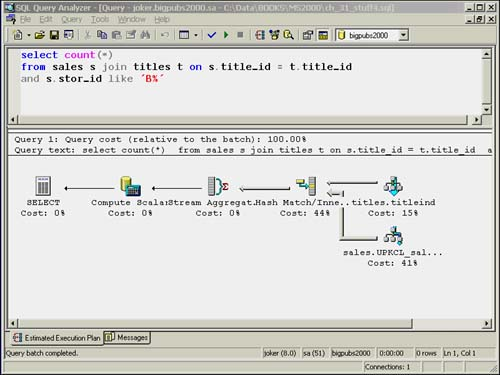The basic hash join algorithm involves separating the two inputs into a "build" input and a "probe" input. The optimizer will always attempt to assign the smaller input as the build input. The hash join scans the build input and creates a hash table. Each row from the build input is inserted into the hash table based on a hash key value, which is computed. The probe input is then scanned one row at a time. A hash key value is computed for each row in the probe, and the hash table is scanned for matches. The hash join is an effective join strategy when dealing with large data volumes and unsorted data inputs.

In a hash join, the keys that are common between the two tables are hashed into a hash bucket using the same hash function. This bucket will usually start out in memory and then move to disk as needed. The type of hashing that occurs depends on the amount of memory required. Hashing is commonly used for inner and outer joins, intersections, unions, and differences. The optimizer often uses hashing for intermediate processing.

Pseudocode for a simple hash join might look like this:

` create an empty hash table  for each row in the input table     read the row     hash the key value     insert the hashed key into the hash bucket for each row in the larger table     read the row     hash the key value     if hashed key value is found in the hash bucket         output hash key and both row identifiers drop the hash table `

Although hashing is useful when no useful indexes are on the tables for a join, the query optimizer still might not choose it as the join strategy if it has a high cost in terms of memory required. If the entire hash table doesn't fit in memory, SQL Server has to split both the build and probe inputs into partitions, each containing a different set of hash keys, and write those partitions out to disk. As each partition is needed, it is brought into memory. This will increase the amount of I/O and general processing time for the query.

To use the hashing strategy efficiently , it is best if the smaller input is used as the build input. If, during execution, SQL Server discovers that the build input is actually larger than the probe input, it might switch the roles of the build and probe input midstream. The optimizer usually doesn't have a problem determining which input is smaller if the statistics are up-to-date on the columns involved in the query. Column-level statistics can help the optimzer determine the estimated number of rows matching a SARG, even if no actual index will be used.

##### Grace Hash Join

If the two inputs are too large to fit into memory for a normal hash join, SQL Server might use a modified method, called the grace hash join. This method partitions the smaller input table (also referred to as the build input) into a number of buckets. The total number of buckets is calculated by determining the bucket size that will fit in memory and dividing it into the number of rows in the table. The larger table (also referred to as the probe input ) is then also partitioned into the same number of buckets. Each bucket from each input can then be read into memory and the matches made. A hybrid join is a join method that uses elements of both a simple in-memory hash and a grace hash.

## NOTE

Hash and merge join strategies can be applied only when the join is an equijoin ; that is, when the join condition compares columns from two inputs with the equality (=) operator. If the join is not based on an equality, for example ”using a BETWEEN clause, nested loop joins are the only strategy that can be employed.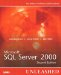Microsoft SQL Server 2000 Unleashed (2nd Edition)
ISBN: 0672324679
EAN: 2147483647
Year: 2002
Pages: 503

Similar book on Amazon